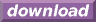#Calcute: freeware calculator

## Common Logarithm (log)

The common logarithm of a number is its logarithm in base 10. For a number x, the common logarithm of x is the power to which 10 must be raised in order to produce x. Calcute implements the common logarithm function with log. The function inverses the effect of raising a number to the power of 10 so that for some number x, log(10x) = x.

```log(2)
0.301029995664
```

Function log is only defined for numbers greater than zero.